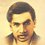# A Nested Radical

Here I present solution to two problems posted on Brilliant: Family of 3's and Infinite sum of reciprocal products and provide a solution to the general expressions.

We know, $\sqrt[a]{n\sqrt[a]{n\sqrt[a]{\cdots}}}=\sqrt[a-1]{n}$

modifying the above one we get,

$\sqrt[a]{n\sqrt[b]{n^{(b-1)/(a-1)}\sqrt[c]{n^{(c-1)/(a-1)}\sqrt[d]{n^{(d-1)/(a-1)}\sqrt[e]{\cdots}}}}}=\sqrt[a-1]{n}$

Putting $a=x+1,b=2x+1,c=3x+1,d=4x+1,e=5x+1,\cdots$ we get,

$\displaystyle \color{#3D99F6} \sqrt[x+1]{n\sqrt[2x+1]{n^2\sqrt[3x+1]{n^3\sqrt[4x+1]{n^4\sqrt[5x+1]{\cdots}}}}}=\sqrt[x]{n}$

Putting $n=3, x=3$ we get, the nested radical as in Family of 3's :

$\sqrt[a_1]{3 \sqrt[a_2]{3^2 \ \sqrt[a_3]{3^3 \ \sqrt[a_4]{\cdots}}}}=\sqrt{3}$ where, $a_n=3n+1$

Now, $\displaystyle \sqrt[x]{n}=\sqrt[x+1]{n\sqrt[2x+1]{n^2\sqrt[3x+1]{n^3\sqrt[4x+1]{n^4\sqrt[5x+1]{\cdots}}}}}$

or,

$n^\frac 1{x} = \left(n \left(n^2 \left(n^3 \left(\cdots \right)^\frac 1{4x+1} \right)^\frac 1{3x+1} \right)^\frac 1{2x+1} \right)^\frac 1{x+1} \\ = \exp \left(\ln n {\color{#3D99F6} \left(\frac 1{x+1} + 2 \cdot \frac 1{x+1} \cdot \frac 1{2x+1} + 3 \cdot \frac 1{x+1} \cdot \frac 1{2x+1} \cdot \frac 1{3x+1} + \cdots \right)} \right) \small \color{#3D99F6} \text{where }\exp (x) = e^x.$

hence,

$\displaystyle \color{#3D99F6} \frac 1{x}=\left(\frac 1{x+1} + 2 \cdot \frac 1{x+1} \cdot \frac 1{2x+1} + 3 \cdot \frac 1{x+1} \cdot \frac 1{2x+1} \cdot \frac 1{3x+1} + \cdots \right)$

Putting $x=3$ we get the infinite series in Infinite sum of reciprocal products:

$\displaystyle \frac 14 + \frac 2{4 \times 7} + \frac 3{4 \times 7 \times 10} + \frac 4{4 \times 7 \times 10 \times 13} + \cdots = \frac 1{3}$

In fact if $a_i \geq 2$ and is an integer then,

$\displaystyle \lim_{s \to \infty}\sum_{k = 1}^s \sum_{i=1}^n \frac k{\prod_{t=1}^k(t\cdot a_i +1)}=\sum_{i=1}^n \frac 1{a_i}$

Using the same nested radicals as above I have derived the following:

• $\displaystyle \sum_{n=1}^{63} \sqrt{n\sqrt{n^2\sqrt{n^3\sqrt{\cdots}}}}=2016$
• $\displaystyle \lim_{m \to \infty} \prod_{j=1}^m \sqrt[a_1]{2 \sqrt[a_2]{2^2 \ \sqrt[a_3]{2^3 \ \sqrt[a_4]{\cdots}}}}=2^\frac{\pi^2}6 \approx 3.127$

$\color{#E81990} \text{Thank You}$Note by Mrigank Shekhar Pathak
3 years, 5 months ago

This discussion board is a place to discuss our Daily Challenges and the math and science related to those challenges. Explanations are more than just a solution — they should explain the steps and thinking strategies that you used to obtain the solution. Comments should further the discussion of math and science.

When posting on Brilliant:

• Use the emojis to react to an explanation, whether you're congratulating a job well done , or just really confused .
• Ask specific questions about the challenge or the steps in somebody's explanation. Well-posed questions can add a lot to the discussion, but posting "I don't understand!" doesn't help anyone.
• Try to contribute something new to the discussion, whether it is an extension, generalization or other idea related to the challenge.
• Stay on topic — we're all here to learn more about math and science, not to hear about your favorite get-rich-quick scheme or current world events.

MarkdownAppears as
*italics* or _italics_ italics
**bold** or __bold__ bold
- bulleted- list
• bulleted
• list
1. numbered2. list
1. numbered
2. list
Note: you must add a full line of space before and after lists for them to show up correctly
paragraph 1paragraph 2

paragraph 1

paragraph 2

[example link](https://brilliant.org)example link
> This is a quote
This is a quote
    # I indented these lines
# 4 spaces, and now they show
# up as a code block.

print "hello world"
# I indented these lines
# 4 spaces, and now they show
# up as a code block.

print "hello world"
MathAppears as
Remember to wrap math in $$ ... $$ or $ ... $ to ensure proper formatting.
2 \times 3 $2 \times 3$
2^{34} $2^{34}$
a_{i-1} $a_{i-1}$
\frac{2}{3} $\frac{2}{3}$
\sqrt{2} $\sqrt{2}$
\sum_{i=1}^3 $\sum_{i=1}^3$
\sin \theta $\sin \theta$
\boxed{123} $\boxed{123}$

## Comments

Sort by:

Top Newest

Amazing! You done amazing work in this post. The global cash prize for the science students is all time amazing and you're offering very well in this post and now you can write my dissertation to manage your task. Keep it up!

- 1 year, 11 months ago

Log in to reply

Good way but should have should have shown more intermediate steps

- 3 years, 3 months ago

Log in to reply

Actually there is an easy way to do it, I will be modifying it soon

- 3 years, 3 months ago

Log in to reply

Wow, I do not understand this at all. Thanks for sharing though.

- 3 years, 5 months ago

Log in to reply

×

Problem Loading...

Note Loading...

Set Loading...